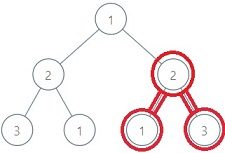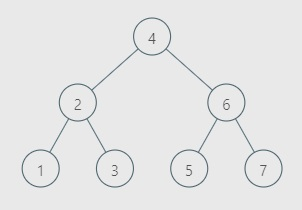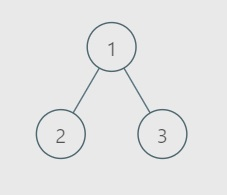1

# Maximum Sum BST

Difficulty: HARDContributed By
Anshul Garg
Avg. time to solve
50 min
Success Rate
55%

Problem Statement

#### You are given a Binary Tree ‘root’. The given Binary Tree may or may not be a Binary Search Tree(BST) itself. Your task is to find the maximum sum of node values of any subtree that is a Binary Search Tree(BST).

##### Binary Search Tree is defined as follows:
``````1) If the left subtree exists it should contain only nodes with values less than the current node's value.

2) If the right subtree exists it should contain only nodes with values greater than the current node's value.

3) Both the left and right subtrees should also be Binary Search Tree.
``````
##### Example :``````For the above binary tree, the BST with the maximum possible sum is marked with RED colour, the sum of this BST is equal to 6.
``````

##### Input Format :
``````The first line contains a single integer ‘T’ denoting the number of test cases, then each test case follows

The first line of each test case contains the elements of the tree in the level order form separated by a single space.

If any node does not have a left or right child, take -1 in its place.

For example, the input for the tree depicted in the below image would be :
````````````1
2 3
4 -1 5 6
-1 7 -1 -1 -1 -1
-1 -1

Explanation :
Level 1 :
The root node of the tree is 1

Level 2 :
Left child of 1 = 2
Right child of 1 = 3

Level 3 :
Left child of 2 = 4
Right child of 2 = null (-1)
Left child of 3 = 5
Right child of 3 = 6

Level 4 :
Left child of 4 = null (-1)
Right child of 4 = 7
Left child of 5 = null (-1)
Right child of 5 = null (-1)
Left child of 6 = null (-1)
Right child of 6 = null (-1)

Level 5 :
Left child of 7 = null (-1)
Right child of 7 = null (-1)

The first not-null node (of the previous level) is treated as the parent of the first two nodes of the current level. The second not-null node (of the previous level) is treated as the parent node for the next two nodes of the current level and so on.

The input ends when all nodes at the last level are null (-1).
``````
##### Output Format :
``````For each test case print the maximum sum possible.

Output for each test case will be printed in a separate line.
``````
##### Note :
``````You are not required to print anything; it has already been taken care of. Just implement the function.
``````
##### Constraints :
``````1 <= T <= 10
1 <= Number Of Nodes <= 5*10^4
0 <= Node.data <= 10^5

Time limit: 1 sec
``````
##### Sample Input 1 :
``````2
4 2 6 1 3 5 7 -1 -1 -1 -1 -1 -1 -1 -1
1 2 3 -1 -1 -1 -1
``````
##### Sample Output 1 :
``````28
3
``````
##### Explanation Of Sample Output 1 :``````For test case 1, the image above shows the input tree:
Given binary tree is itself a BST, also as all the nodes have positive values hence entire tree will be the subtree with maximum sum. Sum of all the node values is: 1 + 2 + 3 + 4 +5 + 6 + 7 = 28
````````````For test case 2, the image above shows the input tree:
The subtree with a single node and node value equal to 3 is the BST with maximum sum.
``````
##### Sample Input 2 :
``````2
2 1 3 -1 -1 -1 -1
1 3 2 2 4 1 3 -1 -1 -1 -1 -1 -1 -1 -1
``````
##### Sample Output 2 :
``````6
9
``````Console